# Viewing the Usage: Overview report in Imagine Math 3+

The Overview section of the Usage report provides class-level averages and individual student totals for time spent using Imagine Math, the number of Math Helps and Live Teachers accessed, and the number of lessons attempted and passed.

Recommendation

Teachers and School Administrators: Sign up for the weekly email. Links to Overview reports grouped by student, by classroom, and by grade level will be sent to you on Sunday nights to remind you to review your data on a regular basis.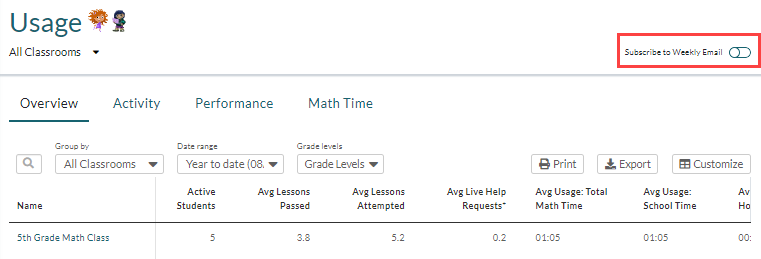Note: District Administrators and Customer Administrators do not have the option to subscribe to the weekly email, but instead have access to the Weekly Progress report from within Imagine Math.

Administrators and Teachers can view the report for all classes and students assigned to them, and the report updates hourly.

To view the Overview section of the Usage reports in Imagine Math 3+:

2. In the Reports section of the left navigation bar, click Usage.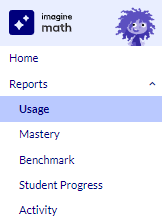3. When it first opens, the Overview tab shows class-level information about usage averages. If desired, use the Group by, Date range, and Grade levels filters to find a particular set of data.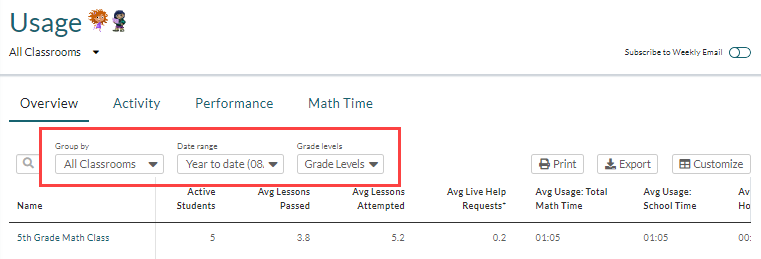The class-level columns that display by default are listed in the table below.
Term/Field Description
Active Students The number of active students in the class. An active student is one who completed at least one math problem.
Avg Lessons Passed The average number of lessons that each student in the class passed. To pass a lesson in Imagine Math 3+, students must either test out of the lesson with an 80% or higher on the Pre-Quiz or score 70% or higher on the Post-Quiz.
Avg Lessons Attempted The average number of lessons that each student in the class attempted. This includes both passed and failed lessons.
Avg Live Help Requests The average number of times each student in the class connected with a Live Teacher. (Imagine Math 3+ students only).
Avg Usage: Total Math Time The class's average usage (in hours and minutes) of Imagine Math 3+ at home and at school combined.
Avg Usage: School Time The class's average usage (in hours and minutes) of Imagine Math 3+ at school. School time is between 7 a.m. and 4 p.m. local time on weekdays.
Avg Usage: Home Time The class's average usage of (in hours and minutes) of Imagine Math 3+ at home. Home time is between 4 p.m. and 7 a.m. on weekdays and all day Saturday and Sunday.
4. If desired, click Customize to add or remove data columns.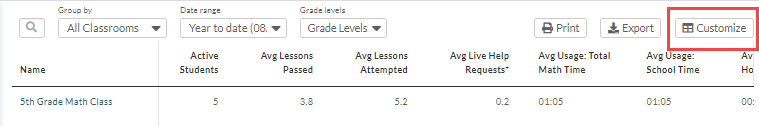At the class level, you can add the following optional columns:

Column Name Description
Avg Problems Attempted The average number of problems attempted by the students in the class.
Avg Math Help Views The average number of times each student accessed the Math Helps. (Imagine Math 3+ students only).
Avg Lesson Pass Rate: Below Grade Level The class's average Lesson Pass Rate (%) for below-grade-level lessons.
Avg Lesson Pass Rate: On Grade Level The class's average Lesson Pass Rate (%) for on-grade-level lessons.
Avg Lessons Passed by Pre-Quiz

The average number of lessons each student passed by scoring 80% or higher on the Pre-Quiz. (Imagine Math 3+ students only).

Avg Pre-Quiz Score The class's average Pre-Quiz score (%). (Imagine Math 3+ students only).
Avg Post-Quiz Score The class's average Post-Quiz score (%). (Imagine Math 3+ students only).
Avg Points Earned The average number of THINK Points each student in the class earned. (Imagine Math 3+ students only).
Avg Tokens Earned The average number of tokens each student in the class earned (Imagine Math PreK-2 students only).
Select the column(s) you wish to add and click Update Columns.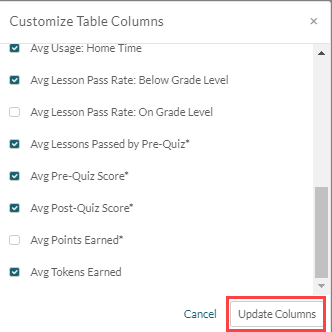5. Click the class name to drill down into individual data for each student in the class.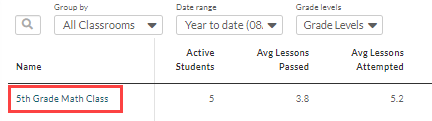The information no longer displays a class average, but rather a total for each student.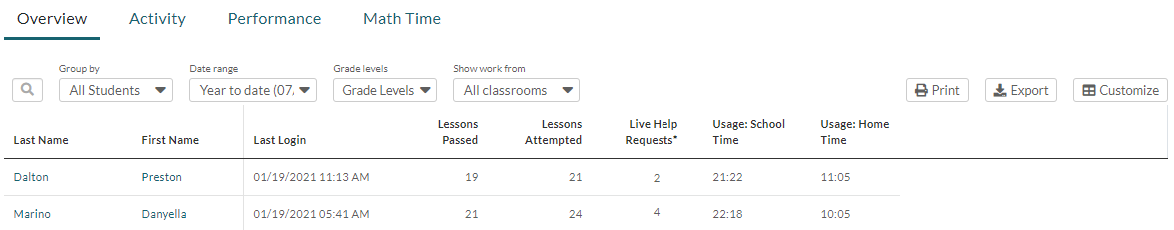Caution

If a student is in more than one class, by default, information on this section of the report displays from all of their classes combined. If you wish to view usage totals and other data only for the currently selected class, select Only this classroom from the Show work from drop-down menu.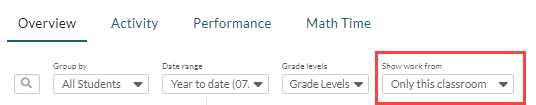The columns that display by default at the student level are listed below:
Column Name Description
Last Login The date/time stamp of the student's most recent login to Imagine Math.
Lessons Passed The total number of lessons that each student in the class passed.
Lessons Attempted The total number of lessons that each student in the class attempted. This includes both passed and failed lessons.
Live Help Requests The total number of times each student in the class connected with a Live Teacher. (Imagine Math 3+ students only).
Usage: School Time In hours and minutes, the total amount of time each student used Imagine Math 3+ at school. School time is between 7 a.m. and 4 p.m. local time on weekdays.
Usage: Home Time In hours and minutes, the total amount of time each student used Imagine Math 3+ at home. Home time is between 4 p.m. and 7 a.m. on weekdays and all day Saturday and Sunday.
6. If desired, click Customize to remove or add columns to the report. The following columns can be added to the student-level report:
Term/Field Description
Student Information Number A unique number assigned to each student when they are rostered into Imagine Math.
Most Recent Performance Level The student's most recent Quantile® Performance Level as measured on their most recent Benchmark assessment. This will be blank if students have only taken Benchmark 1. (Applies to students in all grades except Pre-K).
Benchmark 1 Performance Level The student's Quantile® Performance Level as measured by Benchmark 1. (Applies to students in all grades except Pre-K).
Problems Attempted

The total number of problems the student attempted in all their lessons.

Math Help Views The number of times the student accessed the Live Helps. (Imagine Math 3+ students only).
Usage: Total Math Time The total time the student spent using Imagine Math at home and at school combined.
Lesson Pass Rate: On Grade Level The student's average Lesson Pass Rate (%) for on-grade-level lessons.
Lesson Pass Rate: Below Grade Level The student's average Lesson Pass Rate (%) for below-grade-level lessons.
Lessons Passed by Pre-Quiz The total number of lessons the student passed by testing out via the Pre-Quiz. (Imagine Math 3+ students only).
Avg Pre-Quiz Score The student's average Pre-Quiz score (%). (Imagine Math 3+ students only).
Avg Post-Quiz Score The student's average Post-Quiz score (%). (Imagine Math 3+ students only).
Points Earned The total number of THINK Points each student earned. (Imagine Math 3+ students only).
Tokens Earned The total number of tokens each student earned (Imagine Math PreK-2 students only).
7. Optional: Click Print to open a PDF copy of the report to print or save. Click Export to open a CSV copy of the report to print or save. The file export includes all available columns, regardless of whether or not you added them to the dashboard view.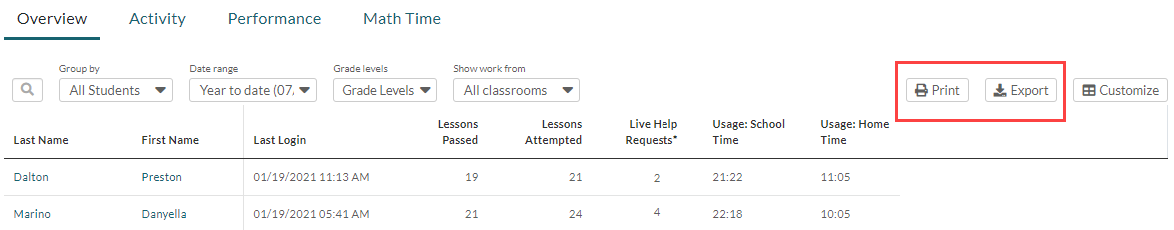8. Optional: Click the name of any student for whom you would like to view a Student Progress report.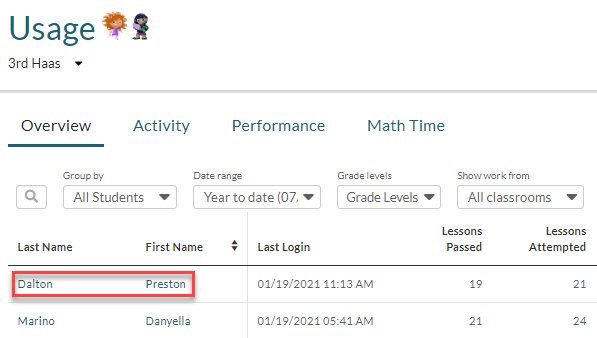Recommendation

Print out an 11" x 17" class progress tracker (in color or black and white), hang it on your classroom wall, and use it to record students' usage of Imagine Math 3+.